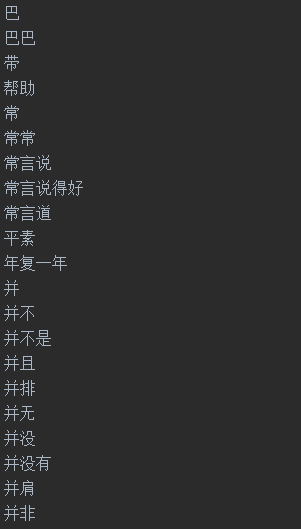**

**

pip install jiebapip install gensim

(.*?) # 匹配URL(.*?)# 匹配content

SamplesGen.py

# -*- coding: utf-8 -*-'''该脚本用于将搜狗语料库新闻语料转化为按照URL作为类别名、content作为内容的txt文件存储'''import osimport re'''生成原始语料文件夹下文件列表'''def listdir(path, list_name):for file in os.listdir(path):file_path = os.path.join(path, file)if os.path.isdir(file_path):listdir(file_path, list_name)else:list_name.append(file_path)'''字符数小于这个数目的content将不被保存'''threh = 30'''获取所有语料'''list_name = []listdir('SogouCS.reduced/',list_name)'''对每个语料'''for path in list_name:print(path)file = open(path, 'rb').read().decode("utf8")'''正则匹配出url和content'''patternURL = re.compile(r'(.*?)', re.S)patternCtt = re.compile(r'(.*?)', re.S)classes = patternURL.findall(file)contents = patternCtt.findall(file)'''# 把所有内容小于30字符的文本全部过滤掉'''for i in range(contents.__len__())[::-1]:if len(contents[i]) < threh:contents.pop(i)classes.pop(i)'''把URL进一步提取出来，只提取出一级url作为类别'''for i in range(classes.__len__()):patternClass = re.compile(r'http://(.*?)/',re.S)classi = patternClass.findall(classes[i])classes[i] = classi'''按照RUL作为类别保存到samples文件夹中'''for i in range(classes.__len__()):file = 'samples/' + classes[i] + '.txt'f = open(file,'a+',encoding='utf-8')f.write(contents[i]+'\n') #加\n换行显示

（1）从samples文件夹中读取所有样本文件

'''生成原始语料文件夹下文件列表'''def listdir(path, list_name):for file in os.listdir(path):file_path = os.path.join(path, file)if os.path.isdir(file_path):listdir(file_path, list_name)else:list_name.append(file_path)list_name = []listdir('samples/', list_name)for path in list_name[0:2]:print(path)file = open(path, 'rb').read().decode('utf-8').split('\n')class_count = 0for text in file:...

（2）分词本文处理的语料均为中文，因此处理中文分词需要使用结巴分词。结巴分词的原理大致是：先使用正则表达式粗略划分，然后基于trie树高速扫描，将每个句子构造有向无环图，使用动态规划查找最大概率路径，基于词频寻找最佳切分方案，最后对于未登录的单词（词表里没有的词语），采用HMM模型，维特比算法划分。分词代码：

content = text# 分词word_list = list(jieba.cut(content, cut_all=False))

（3）去停用词中文中很多词语虽然在文章中大量出现，但对文章分类并没有什么实际意义。比如“只”、“的”、“应该”这样的词语，对它们的计算既浪费空间时间也可能影响最终分类结果。因此需要先建立一个词表，将样本语料分词后出现在该词表中的单词去掉。停用词表按行存储一个单词：去停用词代码：

def get_stop_words():path = "stop_words"file = open(path, 'rb').read().decode('utf-8').split('\r\n')return set(file)def rm_stop_words(word_list):word_list = list(word_list)stop_words = get_stop_words()# 这个很重要，注意每次pop之后总长度是变化的for i in range(word_list.__len__())[::-1]:# 去停用词if word_list[i] in stop_words:word_list.pop(i)#去数字elif word_list[i].isdigit():word_list.pop(i)return word_list

def rm_word_freq_so_little(dictionary, freq_thred):small_freq_ids = [tokenid for tokenid, docfreq in dictionary.dfs.items() if docfreq < freq_thred ]dictionary.filter_tokens(small_freq_ids)dictionary.compactify()

（4）统计词频，生成词袋

for text in file:# 打标签class_count = class_count + 1content = text# 分词word_list = list(jieba.cut(content, cut_all=False))# 去停用词word_list = rm_stop_words(word_list)dictionary.add_documents([word_list])'''转化成词袋gensim包中的dic实际相当于一个mapdoc2bow方法，对没有出现过的词语，在dic中增加该词语如果dic中有该词语，则将该词语序号放到当前word_bow中并且统计该序号单词在该文本中出现了几次'''word_bow = dictionary.doc2bow(word_list)bow.append(word_bow)

（5）生成TF-IDF矩阵TF-IDF相比One-hot编码方式更加合理，它是根据该单词在当前文章中出现的频率和该单词在所有语料中出现的频率评估一个单词的重要性，当一个单词在这篇文章中出现的次数很多的时候，这个词语更加重要；但如果它在所有文章中出现的次数都很多，那么它就显得不那么重要。统计TF-IDF可以使用gensim提供的方法。

# 去除过少单词 ps:可能导致维数不同rm_word_freq_so_little(dictionary,freq_thred)dictionary.save('dicsave.dict')corpora.MmCorpus.serialize('bowsave.mm', bow)tfidf_model = models.TfidfModel(corpus=bow,dictionary=dictionary)with open('tfidf_model.pkl', 'wb') as f2:pickle.dump(tfidf_model, f2)'''训练tf-idf模型'''corpus_tfidf = [tfidf_model[doc] for doc in bow]'''将gensim格式稀疏矩阵转换成可以输入scikit-learn模型格式矩阵'''data = []rows = []cols = []line_count = 0for line in corpus_tfidf:for elem in line:rows.append(line_count)cols.append(elem)data.append(elem)line_count += 1print(line_count)tfidf_matrix = csr_matrix((data,(rows,cols))).toarray()count = 0for ele in tfidf_matrix:# print(ele)# print(count)count = count + 1

dictionary.save('dicsave.dict')corpora.MmCorpus.serialize('bowsave.mm', bow)

（6）输入模型训练/预测

# cut label 1 mil label 0'''生成labels'''labels = np.zeros(sum(labels_count) + 1)for i in range(labels_count):labels[i] = 1

'''分割训练集和测试集'''rarray=np.random.random(size=line_count)x_train = []y_train = []x_test = []y_test = []for i in range(line_count-1):if rarray[i]